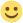• Hi I'm new and i would like to know, how do i calculate out the points of an army, like i have 1000 jags , how many points r the 1000 jags worth ? If possible give me a formula for resource costs or a number of points for each unit ?
ty

• Would be better to ask on the game - it could take a while for a response from admins.

• From FAQ:

- What in-game structures give points for improving the in-game score, and how are they calculated?

In Tentlan, buildings, researches, units and cities give points, they are calculated according to the following rule:

- Each building level gives 10 points;
- each research level - 10 points;
- each unit (incl. damaged units) - 1 point;
- cities: each new city gives 100 points.

Also, the amount of resources spent on buildings/research/units give further points (population and time not counted in):

- 1,000 limestone - 1 point;
- 715 obsidian - 1 point;
- 500 cacao - 1 point;
- 2,500 corn - 1 point.

• Hi I'm new and i would like to know, how do i calculate out the points of an army, like i have 1000 jags , how many points r the 1000 jags worth ? If possible give me a formula for resource costs or a number of points for each unit ?
ty

Hi kekex1337,
As mentioned by PotassiumAstatide you find here (FAQ - 1 General questions: tutorial, bonuses, invite friends...) all the information about the scoring calculation system.

Thank you PotassiumAstatide for your Help

Já fizeste "gosto" na nossa página do facebook?? Acompanha aqui todas as novidades do Tentlan.

• Sorry for the necro hereFor Units, buildings, research, etc. I assume that the points per stone/obsi/cacao is calculated on base cost, not on cost after benefits (like 10% bonus from rituals or diplomacy for units)?

• That's correct, points are always calculated on base costs without bonuses.